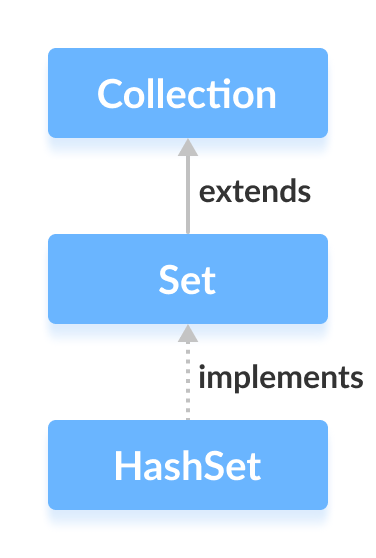Java HashSet Class

# Java HashSet Class

#### In this tutorial, we will learn about the Java HashSet class. We will learn about different hash set methods and operations with the help of examples.

The `HashSet` class of the Java Collections framework provides the functionalities of the hash table data structure.

It implements the Set interface.## Creating a HashSet

In order to create a hash set, we must import the `java.util.HashSet` package first.

Once we import the package, here is how we can create hash sets in Java.

``````// HashSet with 8 capacity and 0.75 load factor
HashSet<Integer> numbers = new HashSet<>(8, 0.75);
``````

Here, we have created a hash set named `numbers`.

Notice, the part new `HashSet<>(8, 0.75)`. Here, the first parameter is capacity, and the second parameter is loadFactor.

• capacity - The capacity of this hash set is 8. Meaning, it can store 8 elements.
• loadFactor - The load factor of this hash set is 0.6. This means, whenever our hash set is filled by 60%, the elements are moved to a new hash table of double the size of the original hash table.

It's possible to create a hash table without defining its capacity and load factor. For example,

``````// HashSet with default capacity and load factor
HashSet<Integer> numbers1 = new HashSet<>();
``````

By default,

• the capacity of the hash set will be 16
• the load factor will be 0.75

## Methods of HashSet

The `HashSet` class provides various methods that allow us to perform various operations on the set.

## Insert Elements to HashSet

• `add()` - inserts the specified element to the set
• `addAll()` - inserts all the elements of the specified collection to the set

For example,

``````import java.util.HashSet;

class Main {
public static void main(String[] args) {
HashSet<Integer> evenNumber = new HashSet<>();

System.out.println("HashSet: " + evenNumber);

HashSet<Integer> numbers = new HashSet<>();

System.out.println("New HashSet: " + numbers);
}
}
``````

Output

```HashSet: [2, 4, 6]
New HashSet: [2, 4, 5, 6]
```

## Access HashSet Elements

To access the elements of a hash set, we can use the `iterator()` method. In order to use this method, we must import the `java.util.Iterator` package. For example,

``````import java.util.HashSet;
import java.util.Iterator;

class Main {
public static void main(String[] args) {
HashSet<Integer> numbers = new HashSet<>();
System.out.println("HashSet: " + numbers);

// Calling iterator() method
Iterator<Integer> iterate = numbers.iterator();
System.out.print("HashSet using Iterator: ");
// Accessing elements
while(iterate.hasNext()) {
System.out.print(iterate.next());
System.out.print(", ");
}
}
}
``````

Output

```HashSet: [2, 5, 6]
HashSet using Iterator: 2, 5, 6,
```

## Remove Elements

• `remove()` - removes the specified element from the set
• `removeAll()` - removes all the elements from the set

For example,

``````import java.util.HashSet;

class Main {
public static void main(String[] args) {
HashSet<Integer> numbers = new HashSet<>();
System.out.println("HashSet: " + numbers);

// Using remove() method
boolean value1 = numbers.remove(5);
System.out.println("Is 5 removed? " + value1);

boolean value2 = numbers.removeAll(numbers);
System.out.println("Are all elements removed? " + value2);
}
}
``````

Output

```HashSet: [2, 5, 6]
Is 5 removed? true
Are all elements removed? true
```

## Set Operations

The various methods of the `HashSet` class can also be used to perform various set operations.

## Union of Sets

Two perform the union between two sets, we can use the `addAll()` method. For example,

``````import java.util.HashSet;

class Main {
public static void main(String[] args) {
HashSet<Integer> evenNumbers = new HashSet<>();
System.out.println("HashSet1: " + evenNumbers);

HashSet<Integer> numbers = new HashSet<>();
System.out.println("HashSet2: " + numbers);

// Union of two set
System.out.println("Union is: " + numbers);
}
}

``````

Output

```HashSet1: [2, 4]
HashSet2: [1, 3]
Union is: [1, 2, 3, 4]
```

## Intersection of Sets

To perform the intersection between two sets, we can use the `retainAll()` method. For example

``````import java.util.HashSet;

class Main {
public static void main(String[] args) {

HashSet<Integer> evenNumbers = new HashSet<>();
System.out.println("HashSet2: " + evenNumbers);

// Intersection of two sets
System.out.println("Intersection is: " + evenNumbers);
}
}
``````

Output

```HashSet1: [2, 3]
HashSet2: [2, 4]
Intersection is: 
```

## Difference of Sets

To calculate the difference between the two sets, we can use the `removeAll()` method. For example,

``````import java.util.HashSet;

class Main {
public static void main(String[] args) {

HashSet<Integer> oddNumbers = new HashSet<>();
System.out.println("HashSet2: " + oddNumbers);

// Difference between HashSet1 and HashSet2
}
}
``````

Output

```HashSet1: [2, 3, 5]
HashSet2: [1, 3, 5]
Difference: 
```

## Subset

To check if a set is a subset of another set or not, we can use the `containsAll()` method. For example,

``````import java.util.HashSet;

class Main {
public static void main(String[] args) {
HashSet<Integer> numbers = new HashSet<>();
System.out.println("HashSet1: " + numbers);

// Check if primeNumbers is a subset of numbers
System.out.println("Is HashSet2 is subset of HashSet1? " + result);
}
}
``````

Output

```HashSet1: [1, 2, 3, 4]
HashSet2: [2, 3]
Is HashSet2 is a subset of HashSet1? true
```

## Other Methods Of HashSet

Method Description
`clone()` Creates a copy of the `HashSet`
`contains()` Searches the `HashSet` for the specified element and returns a boolean result
`isEmpty()` Checks if the `HashSet` is empty
`size()` Returns the size of the `HashSet`
`clear()` Removes all the elements from the `HashSet`

In Java, `HashSet` is commonly used if we have to access elements randomly. It is because elements in a hash table are accessed using hash codes.
`HashSet` cannot contain duplicate elements. Hence, each hash set element has a unique hashcode.